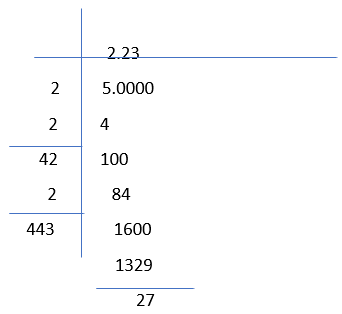# Worksheet on Square Root of Numbers in Decimal and Fractions | Square Root of Fractions and Decimals Worksheet

Worksheet on Square Root of Numbers in Decimal and Fractions contains the questions on the square root of the numbers in decimals and fractions for your practice. To calculate the square root of the numbers in decimals and fractions, we will convert the decimal number to a rational number and then we will find the square root of the by the prime factorization or by the long division method. Here, below we can see the examples on the square root of numbers in decimal and fraction form with solutions.

1. Find the square root of the √1.44 in decimal form?

Solution:

√1.44
Here, we will convert the decimal number to a rational number and then we will find the square root.
= √144/√100
= 1.2

2. Find the square root of √6.25  in decimal form?

Solution:

Given √6.25
To find the square root, we will convert the decimal number to a rational number and then we will find the square root.
= √625/√100
= 2.5

3. Find the square root of √43.56 in Decimal Form?

Solution:

Given √43.56

To find the square root, we will convert the decimal number to a rational number and then we will find the square root.
= √4356/√100
= 6.6

4. Determine √5 up to two places of decimal.

Solution:

Here, we place a dot and zeros to the given square root and then divide into pairs, after that we will choose a number and square the number. So that we will make sure the number is less than or equal to 5 and then we will subtract it. And we will follow the division method as below.5. Estimate √3.6 correct up to two places of decimal?

Solution:

Here, we place a dot and zeros to the given square root and then divide into pairs, after that we will choose a number and square the number. So that we will make sure the number is less than or equal to 3 and then we will subtract it. And we will follow the division method as below.6. Find the Square root of a number √4/25 in fraction form and evaluate the square root of the following numbers in fraction form:

Solution:

Given √4/25
Now we will apply the square root for both numeration and denominator
= √4/√25
on applying the square root, we will get
= 2/5

7. Find the Square root of a number √9/36 in fraction form and evaluate the square root of the following numbers in fraction form?

Solution:

Given √9/36
Now we will apply the square root for both numeration and denominator
= √9/√36
on applying the square root, we will get
= 3/6